Patricia Tries in the Context of Dynamical Systems

Jérémie Bourdon

Greyc, Université de Caen (France)

Algorithms Seminar

March 19, 2001

[summary by Michel Nguyen-The]

A properly typeset version of this document is available in postscript and in pdf.

If some fonts do not look right on your screen, this might be fixed by configuring your browser (see the documentation here).
Abstract
Tries, a generalized form of digital trees, are a data structure widely used in numerous domains: algorithms for searching words, compression, dynamical hashing, ... Their interest and construction lie in the partitioning of a set of words. We present a compact form of tries, called Patricia tries, in which all unary nodes are suppressed (and thus do not intervene in the partitioning). We then study the means of the memory occupation and of the cost of inserting a word for that data structure when words are produced by a probabilistic source for which the dependencies between the emitted symbols can be very important.

## 1  Size and Path Length of Tries and Patricia Tries: Expressions for Expectations

We define the notions of tries and Patricia tries. We find general expressions for the expectations of the size and path length of tries and Patricia tries in the Bernoulli model, valid for any source.

### 1.1  Operations on infinite words

For a finite alphabet S={a1,a2,...,ar}, let S¥ be the set of infinite words on that alphabet, s : S¥®S¥ the map that returns the first letter of a word, and T : S¥®S¥ the shift that returns the first suffix of a word. Let T[a] denote the restriction of T to the set s-1({a}) of words beginning with symbol a and, for a finite prefix w=a1... ak, let T[w] denote the composition T[ak]°T[ak-1]°...°T[a1]. The notations s and T are kept for operators acting on reals which will be used later.

### 1.2  Tries

Definition 1   Let X be a finite set of infinite words produced by the same source. A trie Tr(X) is a structure defined by the following rules:
• (R0) If X=Ø (the empty set), Tr(X) is the empty tree.
• (R1) If X={x}, Tr(X) consists of a single leaf node represented by a square that contains x.
• (R2) If X is of cardinality greater than or equal to 2, Tr(X) is an internal node represented by · to which are attached r subtrees:
Tr(X)= ·, Tr æ
è
T
[a1]X ö
ø
, Tr æ
è
T
[a2]X ö
ø
,... Tr æ
è
T
[ar]X ö
ø
.
The edge that attaches the subtrie Tr(T[aj]X) is labelled by the symbol aj. Notice a little abuse in (R2): if there is no word in X beginning with aj, then T[aj]X is not defined, and we consider that is equal to the empty set. Hence Tr(T[aj]X) is the empty tree, and it is as though there were no subtree corresponding to aj (see Figure 1).

### 1.3  Patricia Tries

A Patricia trie is a trie from which all unary nodes are eliminated.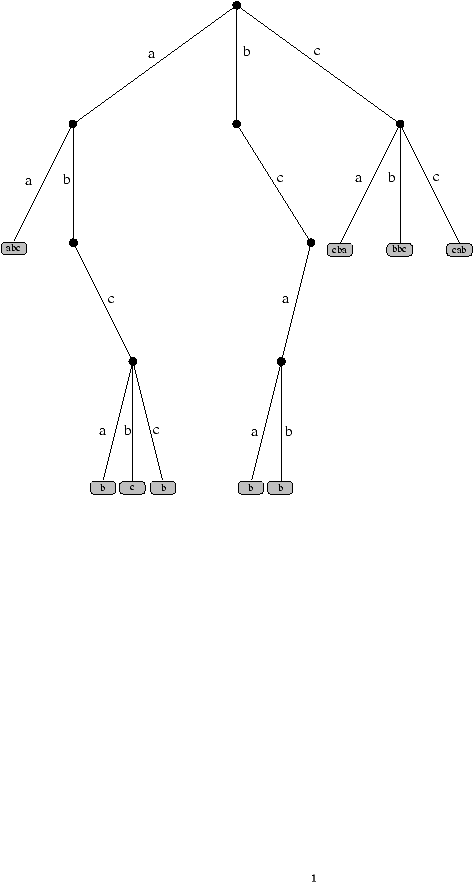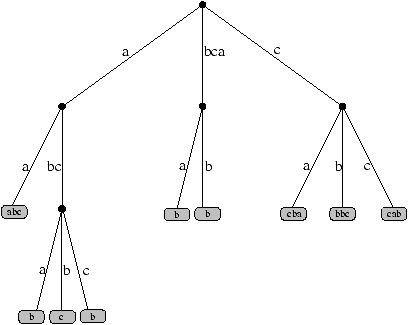Figure 1: Standard trie and corresponding Patricia trie.

Hence with any finite set X of infinite words produced by the same source, we associate a Patricia trie PaTr(X). The first two rules are the same, but the last rule (R'2) is more sophisticated:
• (R'2) If X is of cardinality greater than or equal to 2, we have two cases:
• (R'2,1) if s(X) consists of a single symbol, then PaTr(X) equals PaTr(TX).
• (R'2,2) if s(X) has at least two distinct symbols, PaTr(X) is an internal node generically represented by · to which are attached r subtrees,
PaTr(X)= ·, PaTr æ
è
T
[a1]X ö
ø
, PaTr æ
è
T
[a2]X ö
ø
,··· PaTr æ
è
T
[ar]X ö
ø
.
The edges of the Patricia trie are labelled by words. These words are obtained from the associated trie by concatenating all the labels of the collapsed edges.

The depth of a node in a tree is the number of edges of the path that connects it to the root. The size of a tree is the number of its internal nodes. The path length of a tree is the sum of the depths of all (nonempty) external nodes.

### 1.5  Algebraic analysis of additive parameters

In a standard trie built on the set X={x1, ..., xn}, the structure of a node labelled by a prefix w is a finite string called a slice given by
s
T
[w]X:= æ
è
s
T
[w](x1),···,
s
T
[w](xn) ö
ø
.

An additive parameter g on X is defined by a toll parameter d defined on finite strings and the recursive rule:
g[X]=
ì
ï
í
ï
î
0, if |X|£1,
d[
s
(X)]+
 å mÎS
g[
T
[m]X],
if |X|³2,

Let |s| and #(s) denote the number of symbols of the string s and the number of distinct symbols of s, respectively. The parameters of interest are the size on tries and Patricia tries,
dS(s)= ì
í
î
 1 if |s|³2, 0 otherwise,
dPS(s)= ì
í
î
 1 if #(s)³2, 0 otherwise,
and the internal path length on tries and Patricia tries
dL(s)= ì
í
î
 |s| if |s|³2, 0 otherwise,
dPL(s)= ì
í
î
 |s| if #(s)³2, 0 otherwise.

### 1.6  Expectation of parameters

Let (Pz,S) denote the Poisson model of rate z relative to the source S, and pw the probability that a given infinite word begins with the prefix w. If the cardinality of X is a random Poisson variable of rate z, the length of the slice sT[w]X is also a random Poisson variable of rate zpw. Hence the expectation of parameter g is a sum of expectations of parameter d, E[g;Pz,S]=åwÎS*E[d;Pzpw,Bw].

The expectation of the parameter is given by E[d;Pz,B]=e-z./ u Fd(z,u,p1,···,pr)|u=1, where Fd(z,u,x1,···,xr)=åsÎS*z|s|/|s|!ud(s)x1|s|1··· xr|s|r.

Using algebraic depoissonization , based on the equalities E[Y; Pz]=e-zån³0E[Y; Bn] zn/n! and thus E[Y; Bn]=n![zn]ezE[Y; Pz] zn/n!, one can return to the Bernoulli model. Finally, the expectations of interest are given in Table 1.

 Size of Tr S^(n)=åwÎS*( 1-(1+(n-1)pw)(1-pw)n-1 ) Path Length of Tr L^(n)=åwÎS*npw( 1-(1-pw)n-1 ) Size of PaTr SP^(n)=åwÎS*( 1-(1-pw)n-åiÎS( (1-pw(1-p[i|w]))n-(1-pw)n ) ) Path Length of PaTr LP^(n)=åwÎS*npw( 1-(1-pw)n-1-åiÎSp[i|w]( 1-pw(1-p[i|w]))n-1 )

Table 1: Expectations of size and path length for tries (Tr) and Patricia tries (PaTr).

## 2  Tools for the Asymptotics of the Expectations

### 2.1  Mellin analysis and Dirichlet series

To get asymptotics for the expressions found previously, we first note that they belong to the paradigm of harmonic sums. Their Mellin transforms are given in Table 2, where L(s)=åwÎM*pws and
LS(s)
= -
 å wÎS*
pws -
 å wÎS*
pws
 å iÎS
[ (1-pi|w)s-1 ]

= (s-1)L(s)-s
 å k³2
(-1)k
k!
æ
ç
ç
è
 k-1 Õ i=2
(s-i) ö
÷
÷
ø
[ (s-1)L[k] ]   ,
(1)
LL(s)
=
 å wÎS*
pws
 å iÎS
[ (1-p[i|w])s-1-1 ]

=
 å k³2
(-1)k
(k-1)!
æ
ç
ç
è
 k-1 Õ i=2
(s-i) ö
÷
÷
ø
[ (s-1)L[k] ]  ,
(2)
with L[k](s)=åwÎS*pwsåiÎSpi|wk, for k³1,

 Size of Tr S*(s)=-L(-s)(s+1)G(s) Path Length of Tr L*(s)=-L(-s)G(s+1) Size of PaTr SP*(s)=G(s)LS(-s) Path Length of PaTr LP*(s)=-G(s+1) (L(-s)+LL(-s))

Table 2: Mellin transforms of expectations.

### 2.2  Dynamical sources

We have to restrict ourselves to a class of dynamical sources S (see  for more details and  for its use in a study of standard tries),
• (a) a finite or denumerable alphabet S,
• (b) a topological partition of I:=(0,1) with disjoint open intervals Ia, for aÎS,
• (c) an encoding mapping s which is constant and equal to a on each Ia,
• (d) a shift mapping T whose restriction to to Ia is a real analytic bijection from Ia to I.
Besides, T has to satisfy more precise properties. If we let ha be the local inverse of T restricted to Ia and H be the set H={ ha| aÎS }, then we add properties on bounds of the first derivatives, among which Rényi's condition which plays an important rôle in the study of conditional probabilities. This condition states that, if ha are the local inverse of T, supposed to be locally holomorphic, restricted to Ia, then there exists a constant K that bounds the ratio |ha''(x)/ha'(x)| for all branch ha and all xÎ[0,1]. With each ha, that are only defined on Ia, we associate its analytical extension ha~ to the whole set I.

If M maps xÎ[ 0,1 ] to (s(x),s T(x),s T2(x),...)ÎS¥, T, and s are linked with the previously defined T and s by sMºs and TMº MT.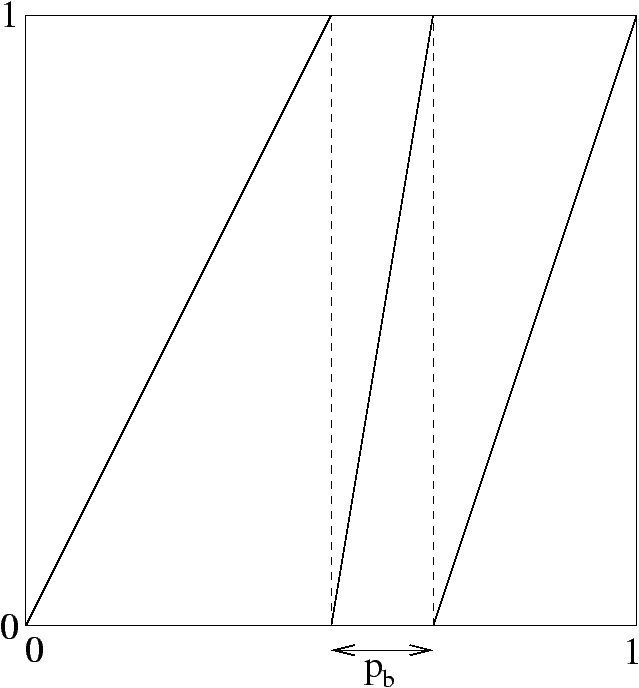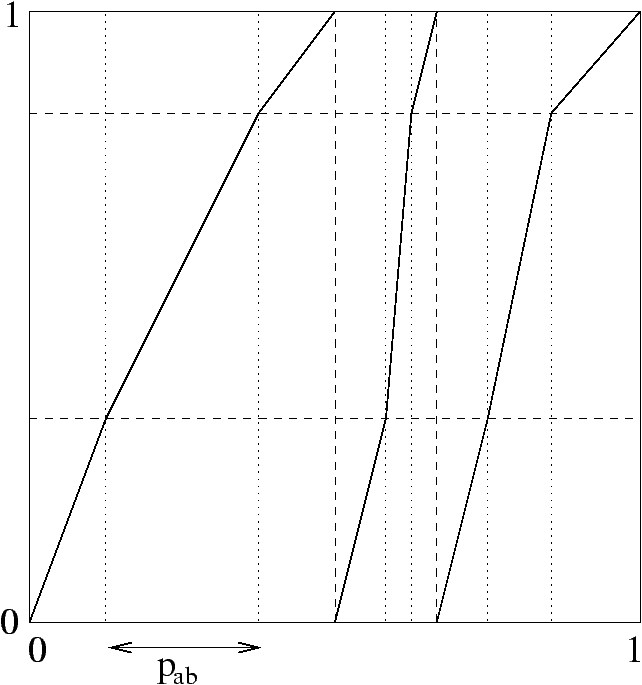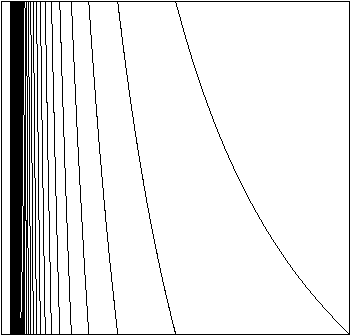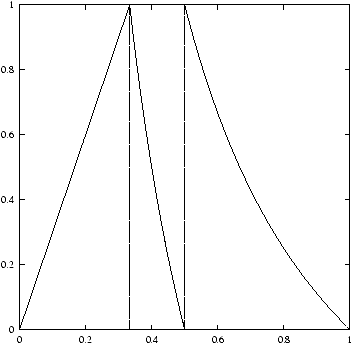Figure 2: Memoryless source, Markov chain of order 1, continued fraction source, heteroclinal source.

Figure 2 displays several types of dynamical sources:

##### Memoryless sources
We have affine branches of slope 1/pa on intervals Ia:=(qa,qa+1), where qa=åi<api.

##### Markov chains
Each Ia of a memoryless source is divided in r intervals Ia,b, bÎS, of length pab=p[b|a]· pa on which T:Ia,b®Ib has slope pa/pab=pb/p[b|a]·1/pa. Notice that when the order d of the Markov chain goes to infinity in a certain sense, one obtains at the limit a source with unbounded memory.
##### Continued fractions
With S=N, Ia:=(1/a+1,1/a), T(x)=1/x-ë1/xû, and s(x)=ë1/xû, corresponding to a continued fraction source, we obtain a source with unbounded memory.

##### Heteroclinal sources
A source for which derivatives in different intervals can be of different signs is called heteroclinal. Otherwise the source is homoclinal, like the sources presented before.

### 2.3  Ruelle operators, multi-secants and prefix probabilities

In the context of dynamical systems, with transformations T of local inverses ha are associated a transfer operator,
G [f](x):=
 å aÎS
| ha'(x) | f° ha(x),
whose interest lies in the following property: if X is a random variable with density function f, then the density of T(X) is G[f]. The Ruelle operator generalizes it by introducing a complex parameter s, interpreted in statistical physics as the temperature:
G s[f](x):=
 å aÎS
 ~ ha
(x)s f° ha(x).

To deal with probabilities of prefixes of words pw and hence with fundamental intervals, we have to replace tangents with secants H[h](x,y):=|h(x)-h(y)/x-y|, leading to a first generalization Gs of the Ruelle operator, acting on functions L of two complex variables:
G s[L](x):=
 å aÎS
 ~ Ha
s[ha](x,y) L ( ha(x),ha(y) ) .
To deal with conditional probabilities, we have to resort to a further generalization Gs of the Ruelle operator involving multisecants instead of secants:
G s[m][L]:=
 å aÎS
Hs[m][ha] L° V[ha],
where the multisecants are defined by Hs[m][h](x,y,z,t)=H[h]s-m(x,y)H[h]m(z,t), and V by V[h](x,y,z,t)=(h(x),h(y),h(z),h(t)).

Let F be the distribution associated with the initial density f of a source (S,f). The probability pw that a word begins with some prefix w is |F(hw(0))-F(hw(1))|. For the special case F=Id, it will be denoted pw*. Let Q:=H[F] be the secant of the initial distribution. Then the quasi-inverses of Gs and Gs[k] are related to Dirichlet series in the following way:
L(s)=
 å wÎM*
pws=(Id-G s)-1[Qs](0,1);    L[k](s)=
 å iÎS
( Id-Gs[k] ) -1 [ Hs[k][F] ] ( 0,1,hi(0),hi(1) ) .

Thanks to a theorem similar to the Perron--Frobenius theorem, we have the decomposition
(Id-Gs)-1 =
l(s)
1-l(s)
Ps+(Id-Ns)-1 ),
and a similar decomposition for the multi-secant operator. We deduce the asymptotics:
 lim s®1
(s-1)(Id-Gs)-1[L](x)=
-1
l'(1)
Y1(x) ó
õ
 1 0
l(tdt),
where Y1(x) is an eigenfunction associated with the dominant eigenvalue and chosen according to a proper normalization, and l is the diagonal mapping of L. We get similar results for the L[m] that also have 1 as pole of order 1, and their respective residues rm are related to the dominant eigenfunctions Y1[m] of the operators G1[m], which allows us to find the singular expansion
L(s)=L(s)~
-1
l'(1)(s-1)
+C(S),
where C(S) is a constant depending on the source S and the initial density f. Using the equalities (1) and (2) we can then get asymptotics for LS(1) and LL(1).

## 3  Results: Asymptotics

### 3.1  General expressions

Let h(S) = -l'(1) = liml®¥åwÎMlpw*|logpw*| be the entropy of fundamental intervals and, besides C(S) encountered before, define the constants
C1(S) =
1-
 å k³2
1
k(k-1)
K[k](S ) =1-
 lim l®¥
 å wÎMl
pw*
 å wÎMl
( 1-p[i|w]* ) | log ( 1-p[i|w]* ) | ,
C2(S) =
 å k³1
1
k
K[k+1](S ) =
 lim l®¥
 å wÎMl
pw*
 å wÎMl
p[i|w]* | log ( 1-p[i|w]* ) | .

For random tries built from n words emitted by a source S, asymptotics of expectations are given in Table 3.

 Size of Tr S(n)»1/h(S)n Path Length of Tr L(n)~1/h(S)nlogn +(C(S)-g/h(S))n Size of PaTr SP(n)»1/h(S) (1-C1(S))n Path Length of PaTr L(n)~1/h(S)nlogn +(C(S)-g+C2(S)/h(S))n

Table 3: Asymptotics of expectations.

### 3.2  Example

For a memoryless source with probabilities {pi}:
h(S) =
 å iÎM
pi | logpi | ,
C(S) =
 å iÎM
pilog2 pi
æ
ç
ç
è
 å iÎM
pilogpi ö
÷
÷
ø
2
,
C1(S) =
1-
 å iÎM
( 1-pi ) | log(1-pi) | ,
C2(S) =
 å iÎM
pi | log(1-pi) | .

Similar formulae are available for Markov chains and continued fraction sources. Simulations are in agreement with theory.

## 4  Conclusion and Open Questions

For the average value of the size, a Patricia trie turns out to be better than a trie, and Rényi's condition is not necessary. For the average value of the path length, there is only a correcting term C2 of order 2, and our proofs made use of Rényi's condition. An open question (see  for details) would be to know whether this correcting term remains valid for sources for which Rényi's condition does not hold, although all the natural sources we are aware of do satisfy that condition.

## References


Bourdon (Jérémie). -- Size and path length of Patricia tries: dynamical sources context. Random Structures & Algorithms, vol. 19, n°3-4, 2001, pp. 289--315. -- Special issue ``Analysis of Algorithms'' dedicated to Don Knuth.


Clément (J.), Flajolet (P.), and Vallée (B.). -- Dynamical sources in information theory: a general analysis of trie structures. Algorithmica, vol. 29, n°1-2, 2001, pp. 307--369.


Jacquet (Philippe) and Szpankowski (Wojciech). -- Analytical de-Poissonization and its applications. Theoretical Computer Science, vol. 201, n°1-2, 1998, pp. 1--62.


Vallée (Brigitte). -- Dynamical sources in information theory: fundamental intervals and word prefixes. Algorithmica, vol. 29, n°1-2, 2001, pp. 262--306.

This document was translated from LATEX by HEVEA.